# Passenger car and truck

From Kutna Hora left at 11:00 clock a truck at 60 km/h. At 12:30 behind him started passenger car at average speed 80 km/h. At what time and how far from Kutna Hora overtake a passenger car truck?

Result

t = 17:00s =  360 km

### Step-by-step explanation:

s = 60(t-11)
s = 80(t-(12+30/60))

s = 60•(t-11)
s = 80•(t-(12+30/60))

s-60t = -660
60s-4800t = -60000

s = 360
t = 17

Our linear equations calculator calculates it.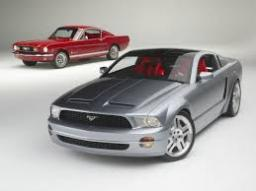Did you find an error or inaccuracy? Feel free to write us. Thank you!Tips to related online calculators
Do you have a linear equation or system of equations and looking for its solution? Or do you have a quadratic equation?
Do you want to convert velocity (speed) units?
Do you want to convert time units like minutes to seconds?

## Related math problems and questions:

• Where and when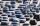The truck left Kremnica at 11:00 h at a speed of 60km/h. At 12:30 h, the passenger car started at an average speed of 80km/h. How many kilometers from Kremnica will the passenger car reach the truck, and when?
• MotionFrom two different locations distant 232 km started against car and bus. The car started at 5:20 with average speed 64 km/h. Bus started at 7:30 with average speed 80 km/h. When they meet? How many kilometers went the bus?
• Truck and car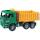At 6h45min, the truck started at a speed of 36 km/h. At 7h30m behind it a passenger car. Find the passenger car's average speed to catch the truck 72 km away from the factory.
• TruckIn 7 hours started from town Krnov truck at speed 40 km/h. Passenger car started against it in 8 hours 30 minutes from the city of Jihlava at speed 70 km/h. Distance between this two cities is 225 km. At what time and at what distance from Krnov this two
• Motorcyclist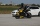From Trutnov, the motorcyclist started at an average speed of 60km/hour. At 12.30hrs the passenger car was started at a speed of 80km/hour. How many hours and at what distance from Trutnov will car catch a motorcycle?
• Truck PRG-BA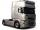Truck driving on the highway from Prague to Bratislava at an average speed of 72 km/h. At the moment when the truck passes 54 kilometers from Prague passenger car departs from Prague, which travels to Bratislava at a speed of 90 km/h. When and where (at w
• Warehouse carsFrom the warehouse started truck at speed 40km/h. After 1hour 30mins started from the same place same direction a car at speed 70 km/h. For how long and at what distance from the warehouse overtake a truck?
• Passenger car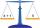Passenger car travels 1 km for 2.3 minutes, a truck during a 50 seconds long. How much is the speed of the truck less?
• Two trains meetFrom A started at 7:15 express train at speed 85 km/h to B. From B started passenger train at 8:30 in the direction to A and at speed 55 km/h. The distance A and B are 386 1/4 km. At what time and at what distance from B the two trains meet?
• Average speed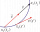The car drove on one section of the highway for half an hour at a speed of 80 km/h. Then he reduced his speed to 60km/h. It went three-quarters of an hour at a speed of 60 km/h. What was the average speed of the car?
• Two cars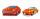From the town A to town B started two cars. The first at 7:00 at average speed 60 km per hour, the second at 10:00 at average speed 100 km per hour. The first car will not stay in B, and on the way back meet the second car at half way from A to B. At what
• Two citiesCities A and B are 200 km away. At 7 o'clock from city A, the car started at an average speed of 80 km/h, and from B at 45 min later, the motorcycle is started at an average speed of 120 km/h. How long will they meet, and at what distance from point A wil
• Motion 2At 10 o'clock started from place A car speed at 90 km/h. Twenty minutes after starting another car from place B at a speed 60 km/h . Distance places A and B is 120 kilometers. What time and where do they meet?
• Znojmo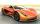From Znojmo to Brno started the truck with a trailer at an average speed 53 km per hour. Against him, 14 minutes later from Brno started the car with an average speed of 1.2-times greater than the truck. How long and how far from Znojmo, they meet if the
• The truck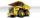The empty truck left the warehouse for goods at 10:00 and arrived at 11:30. At 2:00 pm, they loaded back on the same route. But it was a quarter slower than going to the warehouse. We know that on the way to the warehouse, the car was traveling at a speed
• Two citiesThe distance between cities A and B is 132 km. At 9.00 am, the cyclist started the bike at an average speed of 24 km/h, and at 10.00 am started from the B cyclist at an average speed of 30 km/h. How long and far from A will they both meet?
• Train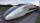A passenger train traveled for 2 hours 74 km. 3.1 hours after its departure started fast train and caught it on 186 km. How many km/h is different its average speeds?